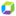cancel
Showing results for
Show  only  | Search instead for
Did you mean:

## Calculation Logic: Percentile Logic AggregationNewcomer

Team,

I wanted to understand how the percentile(90th,95th..) is calculated in Dynatrace?

My Understanding of Percentile Calculation : Assume 90th percentile Pure Path Response Time for 100 transactions .

Step1: Sort the pure path response time for 100 transactions in ascending order.

Step2: Value of the 90th row (90% of the total records(here 100) ) will be the 90th percentile pure path response time saying that 90% of the total records are below this value.

Please provide me the information about how is it calculated in Dynatrace. Thanks.

4 REPLIES 4Dynatrace Pro

Hi Kaushal,

we can't order millions of purepaths as they pass through our analysis engine, so we use the P² algorithm as described e.g. here:

http://www.cs.wustl.edu/~jain/papers/ftp/psqr.pdf

to estimate the percentiles. This also makes it possible to "aggregate" the percentiles for different time frames. Best regards,

PeterThanks Peter.Do we have a restriction in Dynatrace that the percentile calculations will happen only if aggregated for atleast 1 hour+ ?Or it can happen for any selected timeframe?Dynatrace Pro

Hi Kaushal,
Yes, percentile data is calculated hourly, so your smallest timeframe is 1 hour.

Regards,